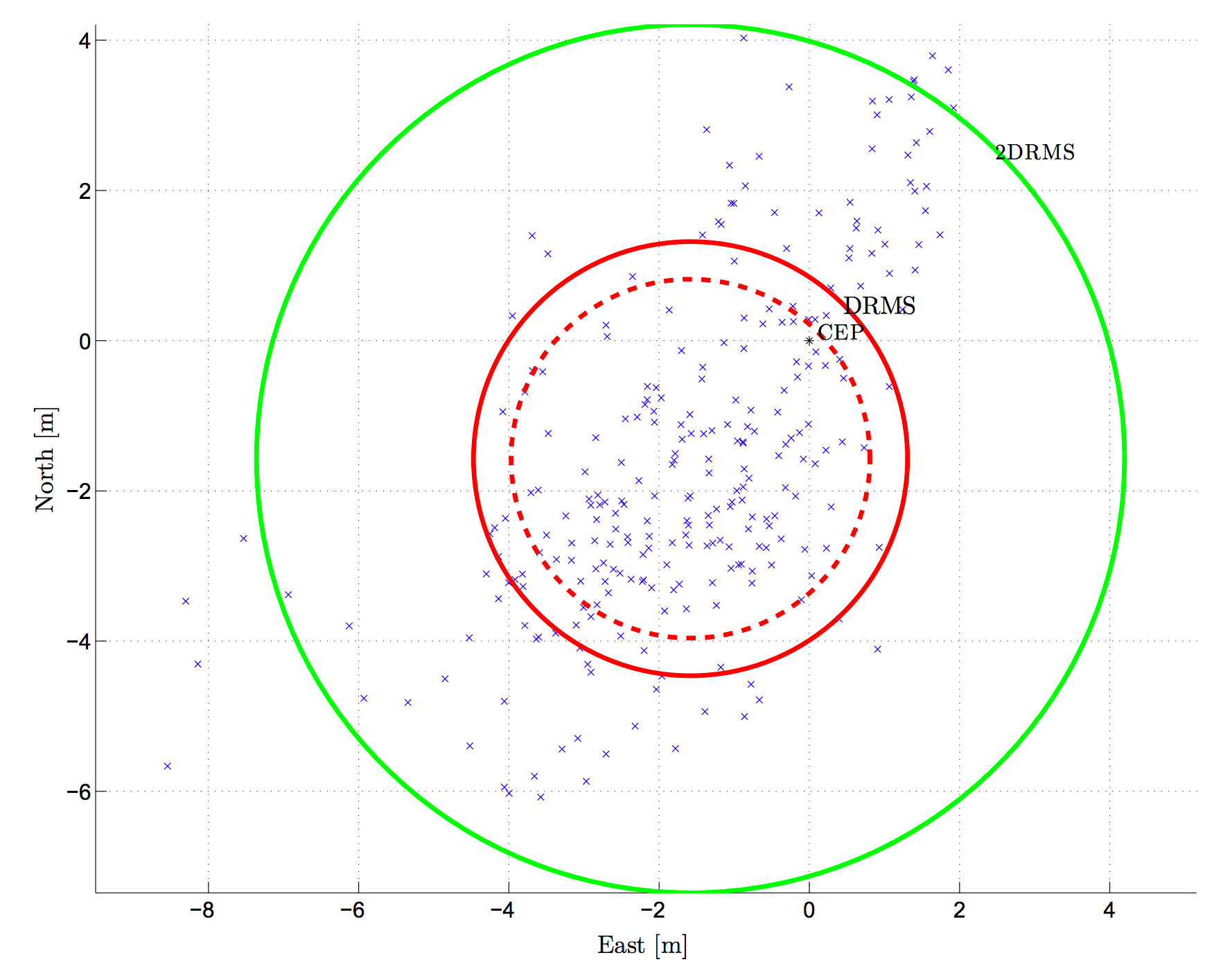# 11.- Repeatability

Repeatability is related to the spread of a measure, also referred to as precision. It refers to how close a position solution is to the mean of all the obtained solutions, in a static location scenario.

The difference between accuracy and precision is shown below:Although the two words precision and accuracy can be synonymous in colloquial use, they are deliberately contrasted in the context of the scientific method. Source: Wikipedia.

The most common precision metrics are defined below:

Measure Formula Confidence region probability
2D 2DRMS $$2\sqrt{\sigma_E^2+\sigma_N^2}$$ 95 %
2D DRMS $$\sqrt{\sigma_E^2+\sigma_N^2}$$ 65 %
2D CEP $$0.62\sigma_N+0.56\sigma_E$$, if $$\frac{\sigma_N}{\sigma_E}>0.3$$ 50 %
3D 99 % SAS $$1.122 \left(\sigma_E^2+\sigma_N^2+\sigma_U^2\right)$$ 99 %
3D 90 % SAS $$0.833 \left(\sigma_E^2+\sigma_N^2+\sigma_U^2\right)$$ 90 %
3D MRSE $$\sqrt{\sigma_E^2+\sigma_N^2+\sigma_U^2}$$ 61 %
3D SEP $$0.51 \left(\sigma_E^2+\sigma_N^2+\sigma_U^2\right)$$ 50 %

which are the same expressions as those defined for accuracy, but now the standard deviations are not referred to a reference value but to the mean of the obtained results:

$\begin{equation} \sigma_{E}^{(precision)} = \sqrt{\frac{1}{L-1}\sum_{l=1}^L \left(E[l] - \hat{E}\right)^2}~, \end{equation}$

where $$\hat{E}=\frac{1}{L}\sum_{l=1}^{L}E[l]$$ is the mean of all the $$E$$ coordinates of the obtained positioning solutions, $$E[l]$$ are the East coordinates of the obtained positioning solutions, and $$L$$ is the number of available position fixes. Similar expressions can be defined for the North and Up coordinates:

$\begin{equation} \sigma_{N}^{(precision)} = \sqrt{\frac{1}{L-1}\sum_{l=1}^L \left(N[l] - \hat{N}\right)^2}~, \end{equation}$

where $$\hat{N}=\frac{1}{L}\sum_{l=1}^{L}N[l]$$, and

$\begin{equation} \sigma_{U}^{(precision)} = \sqrt{\frac{1}{L-1}\sum_{l=1}^L \left(U[l] - \hat{U}\right)^2}~, \end{equation}$

where $$\hat{U}=\frac{1}{L}\sum_{l=1}^{L}U[l]$$.

Example:2D position scatter plot and the circles containing 50%, 65%, and 95% of position fixes (corresponding to the CEP, DRMS, and 2DRMS precision errors, respectively)1.

## Indicators of Repeatability

It follows a list of possible repeatability indicators for a software-defined GNSS receiver:

• Stand-alone receiver’s static positioning precision.
• Differential GNSS static positioning precision.
• Average convergence times to sub-metric precision.

1. C. Fernández-Prades, J. Arribas and P. Closas, Turning a Television into a GNSS Receiver, in Proc. of the 26th International Technical Meeting of The Satellite Division of the Institute of Navigation (ION GNSS+ 2013), Nashville, TN, Sep. 2013, pp. 1492 - 1507.